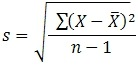How to find standard deviation

Want create site? Find Free WordPress Themes and plugins.

How to find standard deviation?

Related articles about How to find standard deviation

How to subtract fractions

How to find the area of a triangle

How to find the area of a trapezoid

The formula for finding standard deviation is:Where s = sample standard deviation∑ = sum of

Ӿ= sample mean

n = number of scores in sample

People think that finding out the standard deviation is nearly possible plus they face a lot of difficulties with it. It is quite simple and you need to follow the three steps. Are the products at the factory going out of order? How many people are taller than 5 feet 5 inches at the university? How long is it taking for the particle to decay? All of their answers can be found through standard deviation. Let us take an example, if there are five children and they all have different numbers of chocolate bars. One has 5 bars, the other has 6, the other has 7, following one has 8 and the last one has 10. If you are thinking of calculating the mean, you have to add all the numbers and divide by the number of children which is 5.

Calculation: 5 + 6 +7+8+10 = 36

36 ÷ 5 = 7.2

Here the Standard deviation is 1.92354 and the Variance (Standard deviation) is 3.7, the Population Standard deviation is 1.72047 and the Variance (Population Standard deviation) is 2.96. What you can see here that the mean and the standard deviation has a lot of difference. The standard deviation is a graph that looks like it is going up and down. If it was a straight line graph, then there would be no standard deviation. It is represented by sigma “σ” a Greek alphabet.

Let us see how we do it

Step 1: Find out the mean

Take the data you are provided with such as: 5, 10, 15, 20, 30

Mean = Sum of the 5 data ÷ Number of data

= (5+10+15+20+30) ÷ 5

= 80 / 5 = 16

Step 2: Find the variance

Subtract the mean from the given data,

5-16 = -11

10-16 = -6

15-16 = -1

20-16 = 4

30-16 = 14

Step 3: now find the square of the results you got

(-11) ² = 121

(-6) ² =36

(-1) ² = 1

(4) ² = 16

(14) ²= 196

Find the addition of the numbers you got after squaring: 121 + 36 + 1+ 16 +196 = 370. Divide the result with n-1 and n is the number of data you had at the start meaning 5.

370 / (5-1) = 270 / 4 = 92.5

Therefore, the variance is 92.5.

Step 3: To find the standard deviation, carry out the square root of the result you got as variance

√ (92.5)   therefore the result is 9.62.

For this one, variance (Standard deviation) = 92.5, Population Standard deviation=8.60233 and Variance (Population Standard deviation) =74.

Tips:

· While you are just learning it or doing it for the first time, make sure you have all the data and get everything you need like a calculator.

· Write down all the steps and do not skip any part thinking of short-cuts.